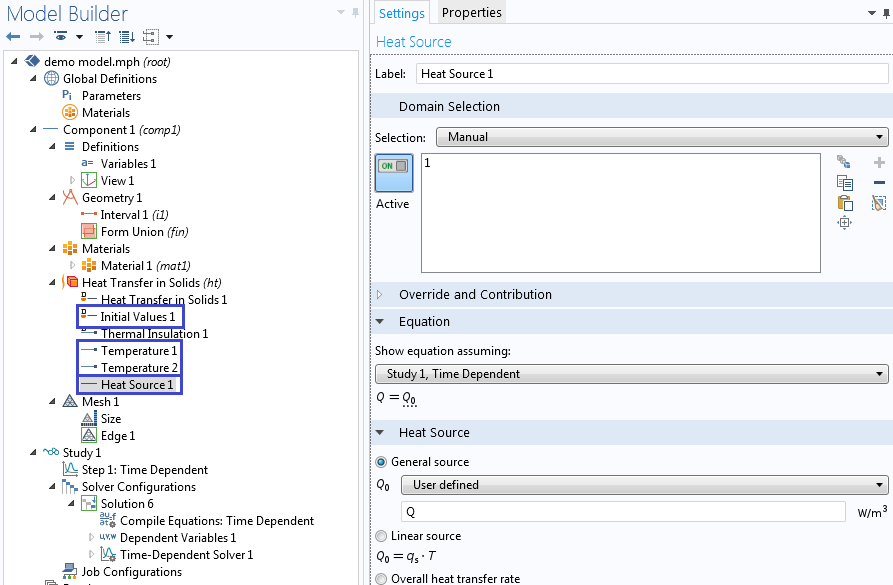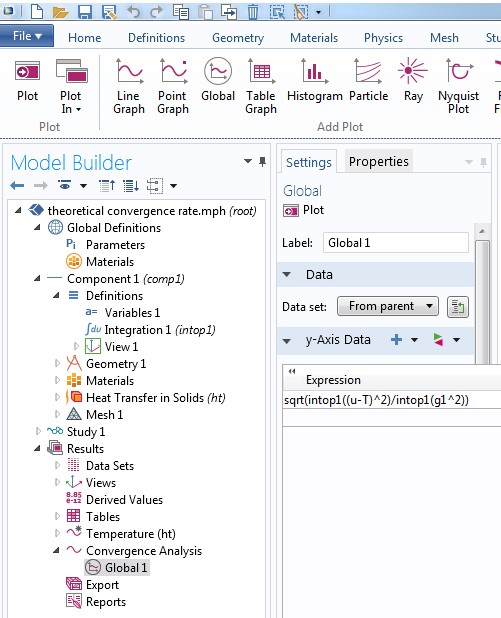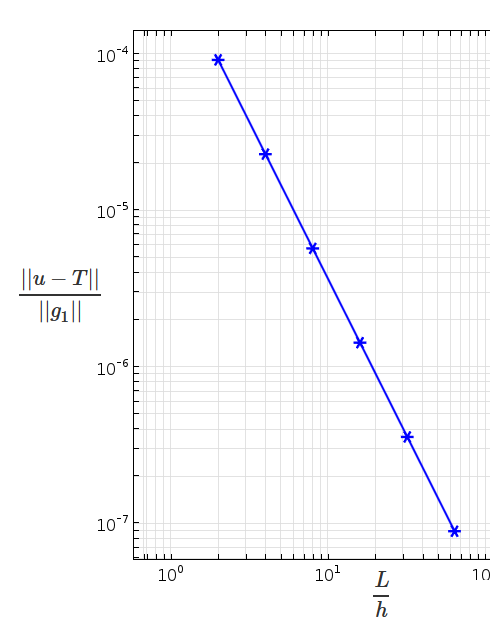# 借助虚构解方法验证仿真

2015年 7月 27日

### 验证与确认

1. 为物理系统建立数学模型。在此我们需考虑会影响观察行为（输出）的所有因素（输入），并假定控制方程。结果通常为一组输入和输出之间的隐式关系式。这往往会是一个包含初始值和边界条件的偏微分方程组，亦称为初边值问题 (IBVP)。
2. 求解数学模型，得到以输入显示函数表示的输出。但在实际操作中，这样的闭式解在大多数问题中并不存在。因此我们使用数值法来获取近似解，并需要计算机的配合来求解大型非线性代数方程组和不等式组。

### 各种验证方法

COMSOL Multiphysics 的 App 库包含许多用到了上述一种或两种方法的验证模型，并按物理领域进行了分类。### 验证一维传热

A_c\rho C_p \frac{\partial T}{\partial t} + \frac{\partial}{\partial x}(-A_ck\frac{\partial T}{\partial x}) = A_cQ, t \in (0,t_f), x \in (0,L)

T(x,0) = f(x)

T(0,t) = g_1(t), \quad T(L,t) = g_2(t).

u(x,t) = 500 K + \frac{x}{L}(\frac{x}{L}-1)\frac{t}{\tau} K,COMSOL Multiphysics 中的符号代数例程可自动求偏导。

f(x) = u(x,0) = 500 K。### 检查代码的各个部分

u(x,t) = 500 K + (\frac{x}{L})^2\frac{t}{\tau} K.

### 收敛速率

||u-u_h||_m \leq C h^{p+1-m}||u||_{p+1},

||e|| = ||u-u_h|| = (\int_{\Omega}(u-u_h)^2d\Omega)^{\frac{1}{2}} \leq C h^{p+1}||u||_{p+1},### 自己动手操作

COMSOL Multiphysics 中内置的符号处理功能使我们可以轻松通过虚构解方法来验证代码，还可用于教学目的。虽然我们会对代码进行广泛测试，但也欢迎用户参与检查。博客介绍了一种用于验证多种物理场接口的通用工具。您也可以使用基于方程的建模或者 COMSOL Multiphysics 中的物理场开发器来验证您的操作。如果您对该技术有任何疑问，欢迎随时与我们联系

### 资源

• 有关虚构解方法及其相关优势和限制的拓展讨论，请参阅桑迪亚国家实验室的报告。该报告详细介绍了一组盲测：一位作者在另一位作者不知情的情况下植入了一系列错误代码，要求其使用本博客中介绍的方法进行扫雷。
• 关于科学计算验证和确认的详细讨论，请参阅
• W. J. Oberkampf and C. J. Roy, Verification and Validation in Scientific Computing, Cambridge University Press, 2010
• 您还可在以下内容中详细了解有限元方法中的标准误差估计
• Thomas J. R. Hughes, The Finite Element Method: Linear Static and Dynamic Finite Element Analysis, Dover Publications, 2000
• B. Daya Reddy, Introductory Functional Analysis: With Applications to Boundary Value Problems and Finite Elements, Springer-Verlag, 1997